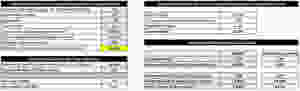# How to avoid bias when unlevering and relevering betas

Edited: Mar 20, 2017

To estimate a firm's WACC, we often have to unlever and relever betas; for example when working with comparable firms that have different capital structures or when assessing the impact of a change in the firm's financing policy on its WACC.

While the process of unlevering and relevering is fairly standard, many practitioners and academics, as well as most textbooks, implement it with overly simplified formulas. These formulas assume that the firm's debt is risk free (put differently, that the beta of debt is 0), which is typically not the case. As a result, the estimated unlevered and relevered betas are systematically biased; and so is the WACC estimate that builds on these betas!

The following example illustrates this point (download the corresponding excel file here). It computes the WACC of a firm that changes its debt ratio from 20% of total assets to 50% of total assets, using standard values for the other ingredients of WACC. The result is rather striking, as you can see at the bottom right of the picture: Using the simplified equation yields a WACC estimate that is more than 1 percentage poing (8.75% vs. 7.65%) higher than the WACC estimate from the "correct" equations.Working with such severely biased WACC estimates will distort investment decisions. In our particular case, the inflated WACC would imply that, under the new capital structure, the firm will reject some positive-NPV projects.

## We have compiled a simple spreadsheet that allows you to use the "correct" equations when unlevering and relevering the cost of capital. The spreadsheet is available for download here.

We hope that this file will be of help for your future estimations of the cost of capital!